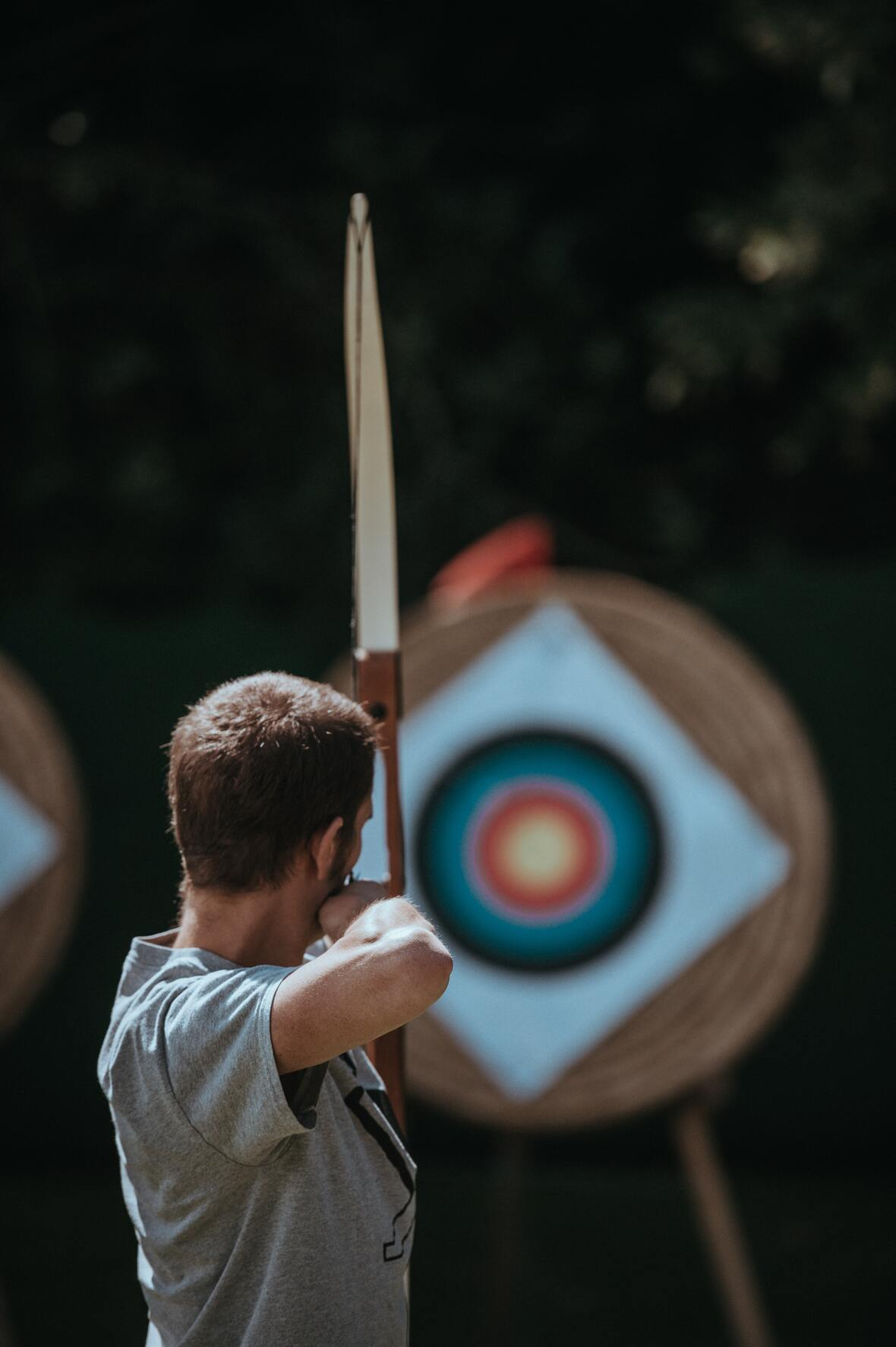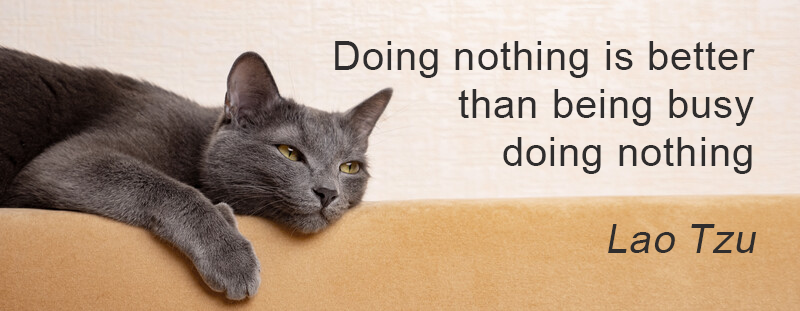Like   Tweet   Pin   +1   in/* styles */ Tips & resources on wellbeing & performance   ||   Issue 41, March 2022
 table div table+table+table div table{width:100%;padding:0}table div table+table+table div table img{width:96.23%;padding:0;float:none}table div table+table+table div table td{width:100%;padding:0 1.88% 18px}/* styles */In this issue I write about Tai Chi and the immune system, breathing and training tips. I've also included a Sports Chi tip and a Lao Tzu quote.

I hope you enjoy reading Good Chi News and welcome any comments you may have.

Regards
Chris Bennett
Chris Chi

 table div table+table+table+table+table div table{width:100%;padding:0}table div table+table+table+table+table div table img{width:96.23%;padding:0;float:none}table div table+table+table+table+table div table td{width:100%;padding:0 1.88% 18px}/* styles */## Tai Chi and the Immune SystemThe focus on the immune system due to Covid is certainly relevant today. This has motivated me to find out more on the positive effect Tai Chi has on the immune system.

I'm researching western and eastern studies on this topic and will share my list with you. I'll include links to each study for those interested in reading the report.

Image courtesy of Kaitlyn Bakervia Unsplash

 table div table+table+table+table+table+table+table+table div table{width:100%;padding:0}table div table+table+table+table+table+table+table+table div table img{width:96.23%;padding:0;float:none}table div table+table+table+table+table+table+table+table div table td{width:100%;padding:0 1.88% 18px}/* styles */## Take a BreathMost of us have heard of the expression 'take a breath', which is good advice when you feel rushed or stressed. In sports, taking a breath when the pressure is on can make the difference between success and failure.

Though the breath needs to be from the abdomen and not the chest to be effective.

In Tai Chi we call this abdominal breathing, sometimes referred to as belly breathing.

Image courtesy Annie Spratt via Unsplash

 table div table+table+table+table+table+table+table+table+table+table+table div table{width:100%;padding:0}table div table+table+table+table+table+table+table+table+table+table+table div table img{width:96.23%;padding:0;float:none}table div table+table+table+table+table+table+table+table+table+table+table div table td{width:100%;padding:0 1.88% 18px}/* styles */## Sports Chi Tip

 /* styles */ Aim for smoothness, roundness and correct alignment when practicing your sports skills.
 table div table+table+table+table+table+table+table+table+table+table+table+table+table+table div table{width:100%;padding:0}table div table+table+table+table+table+table+table+table+table+table+table+table+table+table div table img{width:96.23%;padding:0;float:none}table div table+table+table+table+table+table+table+table+table+table+table+table+table+table div table td{width:100%;padding:0 1.88% 18px}/* styles */## Training When Not TrainingYou will improve your sports skills more when you train away from an official training session. You can do this anytime and anywhere - you don't need equipment or a specific location.

For example, when you are standing or walking practice your Tai Chi alignment for correct posture and efficient movement.

A few seconds, at least three times a week, is all you need to reinforce correct technique so the next time you're at the beginning of an official training session or a game you're ready to perform at your best.

Image courtesy of Sebastien-Goldberg- via Unsplash

 table div table+table+table+table+table+table+table+table+table+table+table+table+table+table+table+table+table div table{width:100%;padding:0}table div table+table+table+table+table+table+table+table+table+table+table+table+table+table+table+table+table div table img{width:96.23%;padding:0;float:none}table div table+table+table+table+table+table+table+table+table+table+table+table+table+table+table+table+table div table td{width:100%;padding:0 1.88% 18px}/* styles */table div table+table+table+table+table+table+table+table+table+table+table+table+table+table+table+table+table+table+table div table{width:100%;padding:0}table div table+table+table+table+table+table+table+table+table+table+table+table+table+table+table+table+table+table+table div table img{width:96.23%;padding:0;float:none}table div table+table+table+table+table+table+table+table+table+table+table+table+table+table+table+table+table+table+table div table td{width:100%;padding:0 1.88% 18px}/* styles *//* styles */
 table div table+table+table+table+table+table+table+table+table+table+table+table+table+table+table+table+table+table+table+table+table div table{width:100%;padding:0}table div table+table+table+table+table+table+table+table+table+table+table+table+table+table+table+table+table+table+table+table+table div table img{width:96.23%;padding:0;float:none}table div table+table+table+table+table+table+table+table+table+table+table+table+table+table+table+table+table+table+table+table+table div table td{width:100%;padding:0 1.88% 18px}/* styles *//* styles */